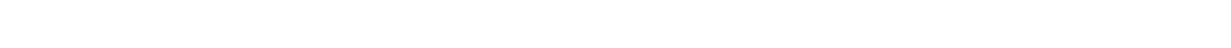# 2.1.3 Relative Atomic Mass Calculations

### Relative Atomic Mass Calculations

• Isotopes are different atoms of the same element that contain the same number of protons and electrons but a different number of neutrons
• These are atoms of the same elements but with different mass numbers
• Because of this, the mass of an element is given as relative atomic mass (Ar) by using the average mass of all of the isotopes
• The relative atomic mass of an element can be calculated by using the percentage abundance values
• The percentage abundance of an isotope is either given or can be read off the mass spectrum
• Firstly, find the mass of 100 atoms by multiplying the percentage abundance by the mass of each isotope
• Secondly, divide by 100 to find the average atomic mass
• For example, if you have two isotopes A and B:#### Worked Example

A sample of oxygen contains the following isotopesWhat is the relative atomic mass of oxygen to 2 dp?

16.00

17.18

16.09

17.00

• Total mass of 100 atoms = (99.76 x 16) + ( 0.04 x 17) + (0.20 x 18) = 1600.44
• Mass of 1 atom = 1600.44 ÷ 100 = 16.0044 = 16.00 (2 dp)

• Here is another example, but this time using a mass spectrum to obtain the information:

#### Worked Example

Calculate the relative atomic mass of boron using its mass spectrum, to 2 dp:• Total mass of 100 atoms = (19.9 x 10) + (80.1 x 11) = 1080.1
• Mass of 1 atom = 1080.1 ÷ 100 = 10.801 = 10.80 (2 dp)

CloseClose